# COMSOL Multiphysics® 5.3 Release Highlights

• Major News in Version 5.3
• Electromagnetics
• Structural & Acoustics
• Fluid & Heat
• Chemical
• Multipurpose
• Interfacing

For users of the Plasma Module, COMSOL Multiphysics® version 5.3 brings a new Global diffusion model to test complex reaction chemistries, an option to approximate the mean electron energy for high-pressure systems, and several new tutorial models. Browse the rest of the new functionality and tutorial models in the Plasma Module below.

### New Application: Boltzmann DC Glow Discharge

This application models a DC glow discharge. The electron energy distribution function (EEDF) and electron transport properties are computed with the Boltzmann Equation, Two-Term Approximation interface. Since input parameters for the Boltzmann Equation, Two-Term Approximation interface, like the ionization degree of the plasma, are not known a priori, an iterative process is performed. A Boltzmann study and a plasma study are computed alternately until the deviation in electron density falls below a user-defined value. As a result, the EEDF at each point of the geometry can be examined.User interface for the Boltzmann DC Glow Discharge example app showing the results of the simulation. User interface for the Boltzmann DC Glow Discharge example app showing the results of the simulation.

Application Library path:
Plasma_Module/Applications/boltzmann_dc_discharge

### Global Modeling for Initial Analyses of Plasma Processes

To facilitate your modeling of plasma processes, a new Global diffusion model now enables you to perform initial analyses of your processes, before optimizing them with more accurate modeling. Global modeling reduces the degrees of freedom for your models through applying ordinary differential equations to your plasma model. This allows complex reaction chemistries to be tested and verified before running space-dependent models, while the reactor geometry, surface chemistry, and feed streams are all still taken into account. To enable global modeling, select the Global option from the Diffusion model drop-down menu, and then choose between the available reactor types:

• Closed reactor
• Constant mass
• Constant pressure

Application Library path for an example showing global modeling:
Plasma_Module/Global_Discharges/chlorine_global_model

### Local Field Approximation

Atmospheric pressure discharges tend to be numerically unstable due to difficulties in computing the mean electron energy. Now, you can avoid computing the mean electron energy through using the Local field approximation option. The transport properties and source coefficients then become functions of the reduced electric field, which is user defined. This approximation is suitable at high pressures, when modeling streamers and coronas.

Application Library path for examples showing the use of Local field approximation:
Plasma_Module/Direct_Current_Discharges/corona_discharge_air_1d
Plasma_Module/Direct_Current_Discharges/streamer_1d

### Automatic Calculation of Electron Mobility

When specifying electron mobility in the Plasma Model Settings window, you can now specify the transport properties to be automatically computed from a list of electron impact reactions.

Application Library paths for examples using the From electron impact reactions option:
Plasma_Module/Direct_Current_Discharges/argon_dbd_1d
Plasma_Module/Inductively_Coupled_Plasmas/electrodeless_lamp

### Unit Support for Tables in the Plasma Interface

It is now possible to change the units of the data in the two columns of a table in the plasma interfaces. Units are now available in the following features.

#### Electron Impact Reactions

• When Specify reaction using is set to Cross section data, a table of Electron energy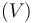and Collision cross section data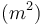is available
• When Specify reaction using is set to Use lookup table and Rate constant form is set to Rate coefficient, a table of Mean electron energyand Rate coefficient data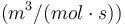is available
• When Specify reaction using is set to Use lookup table and Rate constant form is set to Townsend coefficient, a table of Mean electron energyand Townsend coefficient datais available

#### Species

• When the Species is an Ion and the Mobility and Diffusivity Expressions are set to Specify mobility, compute diffusivity, then the Ion mobility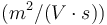can be specified in terms of the Electric field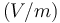• When the Species is an Ion and the Mobility and Diffusivity Expressions are set to Specify mobility, compute diffusivity, then the Ion mobilitycan be specified in terms of the Reduced electric field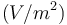#### Plasma Model

When the interface property Use reduced electron transport properties is inactive and when Electron transport properties is set to Use lookup tables, then four tables are available for:

• Electron mobility, which has two columns for the Mean electron energyand the Electron mobility• Electron diffusivity, which has two columns for the Mean electron energyand the Electron diffusivity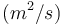• Electron energy diffusivity, which has two columns for the Mean electron energyand the Electron energy diffusivity• Electron energy mobility, which has two columns for the Mean electron energyand the Electron energy mobilityWhen the interface property Use reduced electron transport properties is active and when Electron transport properties is set to Use lookup tables, then four tables are available for:

• Reduced electron mobility, which has two columns for the Mean electron energyand the Reduced electron mobility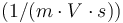• Reduced electron diffusivity, which has two columns for the Mean electron energyand the Reduced electron diffusivity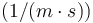• Reduced electron energy diffusivity, which has two columns for the Mean electron energyand the Reduced electron energy diffusivity• Reduced electron energy mobility, which has two columns for the Mean electron energyand the Reduced electron energy mobilityTwo new models are available for computing ion mobility. The Dalgarno model requires specification of the ion polarizability and is valid when the electric field is low (the ion drift velocity is much less than the ion thermal velocity). The High field model is valid when the ion drift velocity is much greater than the thermal velocity.

### New Tutorial Model: Atmospheric Pressure Corona Discharge in Air

This tutorial model presents a study of a coaxial DC corona discharge in dry air at atmospheric pressure. The dimensions and operation conditions are similar to ones found in electrostatic precipitators with wire-to-plate configurations. The inner wire electrode has a 100-mm radius and the gap between electrodes is 10 cm.

The model solves the electron and ion continuity and momentum equations in the drift-diffusion approximation, self-consistently coupled with Poisson’s equation. The local field approximation is used, which means that transport and source coefficients are assumed to be well parameterized through the reduced electric field.

The simulations presented are for steady-state regimes, with a sustained discharge and 10's of kV applied to the inner electrode while the exterior electrode is grounded. Emphasis is placed on the charged particles' creation and transport and how that translates into the current-voltage characteristic of the discharge.Electron, positive ion, and negative ion densities in a corona discharge with a simplified air chemistry. Electron, positive ion, and negative ion densities in a corona discharge with a simplified air chemistry.

Application Library path:
Plasma_Module/Direct_Current_Discharges/corona_discharge_air_1d

### New Tutorial Model: Negative Streamer in Nitrogen in 1D

Streamers are transient filamentary electric discharges that can develop in a nonconducting background in the presence of an intense electric field. These discharges can attain high electron number density and consequently, a high concentration of chemical active species that are relevant for numerous applications. Industrial applications include ozone production, pollution control, and surface processing.

The propagation of streamers is driven by very nonlinear dynamics that involve very steep density gradients and high space-charge density distributed in very thin layers. This tutorial model presents a study of a negative streamer in atmospheric pressure nitrogen in a constant electric field of -100 kV/cm. The model is one-dimensional and describes the transient behavior of an initial electron seed from electron growth in an unperturbed electric field to streamer propagation.Spatial distribution of the electron (colored solid lines) and ion number density (black dashed lines) for four time instants during streamer propagation. Spatial distribution of the electron (colored solid lines) and ion number density (black dashed lines) for four time instants during streamer propagation.

Application Library path:
Plasma_Module/Direct_Current_Discharges/streamer_1d

### New Tutorial Model: Chlorine Discharge

Plasma discharges containing chlorine are commonly used to etch semiconductors and metals in microelectronics fabrication.

This tutorial model studies chlorine plasma discharges using a global (volume-averaged) diffusion model. These can run simulations in a fraction of the time it would take for space-dependent models. This makes them a good choice to study large reaction sets and extended parameter regions.

The Chlorine Discharge model explores absorbed powers from 50 to 600 W, working with pressures from 1 to 100 mTorr. Model results of several relevant quantities such as electron density, electron temperature, and atomic chlorine density are in good agreement with measurements performed in inductively coupled plasma reactors, found in the literature.Evolution of the chlorine species and electron density in the reactor using a global diffusion model. Evolution of the chlorine species and electron density in the reactor using a global diffusion model.

Application Library path:
Plasma_Module/Global_Discharges/chlorine_global_model

### New Tutorial Model: Surface Chemistry

Surface chemistry is often an overlooked aspect of reacting flow modeling. This tutorial model shows how surface reactions and species can be added to study processes like chemical vapor deposition (CVD). The tutorial then models silicon growth on a wafer.

Initially, the example uses a global model to investigate a broad region of parameters with complex chemistry. Then, a space-dependent model is set up and run. Careful attention is paid to the overall mass balance in the system while the difference between the mass-averaged velocity and diffusion velocity is explored. The model demonstrates that the total mass and molar concentration in the system is conserved. Finally, the height of the deposited silicon is studied as a function of time.Growth of the deposited silicon on a boundary for the global model (right) and the space-dependent model (left). The x-axis represents the space dimension (m), the y-axis represents time (s), and the z-axis represents the growth height (Å). Since this is a closed reactor with a uniform distribution of the species, the agreement between the two methods is very good.

Growth of the deposited silicon on a boundary for the global model (right) and the space-dependent model (left). The x-axis represents the space dimension (m), the y-axis represents time (s), and the z-axis represents the growth height (Å). Since this is a closed reactor with a uniform distribution of the species, the agreement between the two methods is very good.

Application Library path:
Plasma_Module/Chemical_Vapor_Deposition/surface_chemistry_tutorial

### New Tutorial Model: Microwave Microplasma

Plasmas sustained in microscale discharge gaps are able to operate at high pressures (1 atm) with high electron number density (1E20 m^-3) and power density (1E9 W/m^3), while maintaining a relatively cool heavy-particle temperature. This tutorial model simulates an atmospheric-pressure argon plasma sustained by a time-varying electric excitation in the microwave range. The model is one-dimensional in the direction of the applied field and describes the spatial and temporal evolution of several macroscopic properties of the plasma.Evolution of the log of the electron density during the 500th RF cycle. The y-axis in this plot represents time multiplied by the excitation frequency.

Evolution of the log of the electron density during the 500th RF cycle. The y-axis in this plot represents time multiplied by the excitation frequency.

Application Library path:
Plasma_Module/Capacitively_Coupled_Plasmas/microwave_microplasma